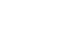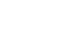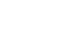## KCSE 2014 Physics Paper 3 Questions with Marking Scheme

QUESTION 1

You are provided with the following:

• a voltmeter
• a milliammeter
• a micrometer screw gauge (to be shared)
• a stopwatch
• a centre zero galvanometer
• a switch
• ten connecting wires (at least with a crocodile clip on one end)
• a resistance wire mounted on a millimetre scalelabeled AB
• a resistance wire labeled P
• a resistance wire labeled Q
• a capacitor labeled C
• a metre rule or half metre rule
• two dry cells and a cell holder
• a carbon resistor labeled R

Proceed as follows:

PART A

1. Using the micrometer screw gauge provided, measure the diameter:
1. D of wire P.
D = _________________ (1 mark)
2. d of wire Q
d = _________________ (1 mark)

2. Determine C1 the value of the ratio D/d
C1 = __________________ (1 mark)

1. Set up the circuit as shown in figure 1. (Ensure that each of the wires P and Q is 50 cm long)Close the switch. Using the clip at the the free end from the wire from the galvanometer, tap wire AB near end A and observe the defection in the galvanometer.

2. Then tap the wire near end B and then observe the deflection in the galvanometer.
3. Now tap the wire AB at various points between A and B to obtain a point K where there i no deflection in the galvanometer.
1. Determine the length L1, the distance from A to K.
L1 = ________________ (1 mark)
2. Determine the length L2, the distance from B to K.
L2 = ______________ (1 mark)

1. Given that resistance RQ of Q is 9.0 ohms, determine the resistance RP of P using the expression: (2 marks)2. Determine the value of C2 given that, (2 marks)3. Compare the value of C1 (in part (b)) with that of C2. (1 mark)
PART B

3. Set up the circuit shown in figure 2. S and T are crocodile clips.1. Charge the capacitor C by connecting the crocodile clip to S. Record the reading of the voltmeter, V
V = _____________ (1 mark)

2. Calculate the value of current of Io , given that Io = V/R (where R = 4.7 x 103 Ω) (3 marks)

1. Discharge the capacitor by by disconnecting the crocodile clip from S and connecting it to T. Observe and record the highest reading of the milliammeter I1. (This is the current at t0 = 0).
(You may have to repeat the process to obtain an accurate value.)
I1 = _______________ (1 mark)

2. Recharge the capacitor by connecting the crocodile clip to S.

3. Discharge the capacitor and at the same time start the stop watch to measure the time t1 taken for the current to decrease to the half the value of I1  ie (1/2I1)
t1 = ______________ (1 mark)

1. Recharge the capacitor and repeat the procedure in f(iii) to measure the time t2 taken for the the current to decrease to one tenth of the value of I1  i.e (1/10I1)
t2 = _____________ (1 mark)
2. Use the values of the currents corresponding times to draw a graph of current I (y axis) on the grid provided. (3 marks)QUESTION 2
You are provided with the following:

• a stand boss and clamp
• two wooden blocks
• a stopwatch
• a half metre rule or metre rule
• a metallic rod
• a bare copper wire labelled M attached to a crocodile clip
• a bare copper wire labeled N attached to a crocodile clip

Proceed as follows:
1. Clamp wire M between the wooden blocks so that the length l of the wire between the wooden blocks and the point of its attachment on the crocodile clip is 5 cm. Clamp the metallic rod at its mid-point using the crocodile clip attached to wire M.

2. Displace the rod through a small angle in a horizontal plane aboutits mid point so thst when released, it oscillates in the same plane. Record the time t for 10 oscilations and determine the period T in Table 1.

3. Repeat part (b) for the other lengths of wire M shown in Table 1.

4. Complete Table 1. (6 marks)5. Plot a graph l (y axis)against T2.6. Determine the gradient of the graph, S. (3 marks)

7. Now replace wire M with wire N in the set up.
1. For l = 20 cm, displace the rod through a small angle in the horizontal plane and measure the time tN for ten oscillations.
tN = __________ (1 mark)
2. Determine the period TN = ____________ (1 mark)
3. Calculate TN2 (1 mark)
4. Determine the value of H given that(1 mark)
5. Calculate the value of H/S (2 marks)## MARKING SCHEME

QUESTION ONE PART A

1.
1. D = 0.38 mm ± 0.02 (1 mark)
2. d = 0.28 mm ± 0.05 (1 mark)
2. C1 = D = 0.38 = 1.357 (1 mark)
d    0.28
3.
1. L1 = 38.5 cm (1 mark)
L2 = 61.5 cm (1 mark)
(l1 < l2)
Rp = 38.5
9      61.5
therefore: Rp = 5.63Ω
C2 = √  9
5.63
= 1.264   (2 marks)
2. C1 and C2 are nearly equal (to the nearest whole number).(1 mark)

QUESTION ONE PART B

V = 3.1 volts ± 0.1
I0 = V  =      3.1      A
R      4.7  × 103
= 0.659 mA   (3 marks)
I1 = 0.63 mA
For = I1
2
t1 = 3.9 s(1 mark)
For = I1
10
t2 = 13.5 s (1 mark)

 I 0.5 0.25 0.05 t 0 3.6 12.5(3 marks)

QUESTION TWO
(d)

 l(cm) 5 10 15 20 25 30 t(s) 20.1 26.3 31.2 33 39.6 43.4 T(s) 2.01 2.63 3.12 3.3 3.96 4.34 T2(S2) 4.04 6.92 9.73 10.89 15.68 19.84

(6 marks)
(e) Graph.(5 marks)
16
= 0.20 cm/s2
16
= 0.015625 ms-2   (3 marks)
(g) lN = 20 cm = 0.2 m

1. tN = 52.0
2. TN = 5.2
3. TN2 = 27.04(1 mark)
H =   0.2    = 0.007396 (1 mark)
27 04
4. H = 0.007396
S    0.015625
= 0.4737     (2 marks)

• ✔ To read offline at any time.
• ✔ To Print at your convenience
• ✔ Share Easily with Friends / Students